The Japanese Bonsai specialist
Direct order Contact Help / Services NewsletterSaxifraga fortunei dancing pixies

Saxifraga fortunei dancing pixies daimonjiso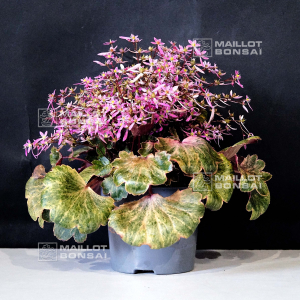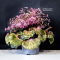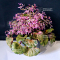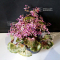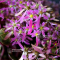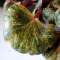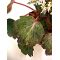ref. : 11314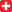11,50

Available quantity : 2Order

Description

Small vivacious plant to the brilliant thick leaves of Japanese origin. Flowering of mid October to end November according to the varieties and the arrival of the cold weather. Flowers doing recall the shape of the kanji dai from where does its name come[Daimonjisô]. Abundant flowering in panicles on shaft of 15 /25 centimeters of height. Inflorescence composed of 3 oval superior petals of small dimension and 2 inferior a lot stretched out. Height of 10 has 25 cm according to varieties.

Culture: Ask for an exhibition mid-shaded to shady in soil slightly acidic cool humifère and drained well. Not to let water stagnate to the foot of the plant nor the heaps of dead leaves that would encourage the rot of the collar. Can be cultivated in pot (culture in cool piece as the cyclamen hivernage out frost) in kusamono to accompany the bonsaï, but also in plates, on low walls and in full earth. This species resists has temperatures of -15 to-18 degrees on soil draining us cultivate it since more of 10 years in our nursery of Raising with success. The saxifrages loses in winter their leaves that start again in the spring.

#saxifrages 4 #leaves 3.6 #according 2.9 #saxifraga 2.9 #flowering 2.9 #varieties 2.9 #fortunei 2.8 #culture 2.7 #dancing 2.7 #height 2.6

Formule
(( ROUND((CHAR_LENGTH(b.article_nom)-CHAR_LENGTH(REPLACE(b.article_nom, 'leaves', '')))/LENGTH('leaves')) + ROUND((CHAR_LENGTH(b.article_description)-CHAR_LENGTH(REPLACE(b.article_description, 'leaves', '')))/LENGTH('leaves')) ) * 3.6) + (( ROUND((CHAR_LENGTH(b.article_nom)-CHAR_LENGTH(REPLACE(b.article_nom, 'according', '')))/LENGTH('according')) + ROUND((CHAR_LENGTH(b.article_description)-CHAR_LENGTH(REPLACE(b.article_description, 'according', '')))/LENGTH('according')) ) * 2.9) + (( ROUND((CHAR_LENGTH(b.article_nom)-CHAR_LENGTH(REPLACE(b.article_nom, 'saxifraga', '')))/LENGTH('saxifraga')) + ROUND((CHAR_LENGTH(b.article_description)-CHAR_LENGTH(REPLACE(b.article_description, 'saxifraga', '')))/LENGTH('saxifraga')) ) * 2.9) + (( ROUND((CHAR_LENGTH(b.article_nom)-CHAR_LENGTH(REPLACE(b.article_nom, 'flowering', '')))/LENGTH('flowering')) + ROUND((CHAR_LENGTH(b.article_description)-CHAR_LENGTH(REPLACE(b.article_description, 'flowering', '')))/LENGTH('flowering')) ) * 2.9) + (( ROUND((CHAR_LENGTH(b.article_nom)-CHAR_LENGTH(REPLACE(b.article_nom, 'varieties', '')))/LENGTH('varieties')) + ROUND((CHAR_LENGTH(b.article_description)-CHAR_LENGTH(REPLACE(b.article_description, 'varieties', '')))/LENGTH('varieties')) ) * 2.9) + (( ROUND((CHAR_LENGTH(b.article_nom)-CHAR_LENGTH(REPLACE(b.article_nom, 'fortunei', '')))/LENGTH('fortunei')) + ROUND((CHAR_LENGTH(b.article_description)-CHAR_LENGTH(REPLACE(b.article_description, 'fortunei', '')))/LENGTH('fortunei')) ) * 2.8) + (( ROUND((CHAR_LENGTH(b.article_nom)-CHAR_LENGTH(REPLACE(b.article_nom, 'dancing', '')))/LENGTH('dancing')) + ROUND((CHAR_LENGTH(b.article_description)-CHAR_LENGTH(REPLACE(b.article_description, 'dancing', '')))/LENGTH('dancing')) ) * 2.7) + (( ROUND((CHAR_LENGTH(b.article_nom)-CHAR_LENGTH(REPLACE(b.article_nom, 'culture', '')))/LENGTH('culture')) + ROUND((CHAR_LENGTH(b.article_description)-CHAR_LENGTH(REPLACE(b.article_description, 'culture', '')))/LENGTH('culture')) ) * 2.7) + (( ROUND((CHAR_LENGTH(b.article_nom)-CHAR_LENGTH(REPLACE(b.article_nom, 'height', '')))/LENGTH('height')) + ROUND((CHAR_LENGTH(b.article_description)-CHAR_LENGTH(REPLACE(b.article_description, 'height', '')))/LENGTH('height')) ) * 2.6) + (( ROUND((CHAR_LENGTH(b.article_nom)-CHAR_LENGTH(REPLACE(b.article_nom, 'pixies', '')))/LENGTH('pixies')) + ROUND((CHAR_LENGTH(b.article_description)-CHAR_LENGTH(REPLACE(b.article_description, 'pixies', '')))/LENGTH('pixies')) ) * 2.6)

Secure paymentDelivery

Our logistic partners :04 74 55 23 48
Pépinière MAILLOT-BONSAÏ
Le Bois Frazy
01990 RELEVANT - FRANCE
on appointment